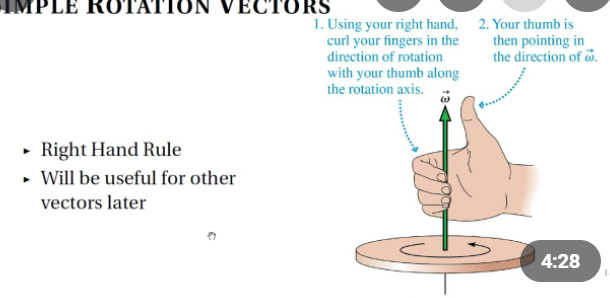# Problem with finding degree between vectors

Hello,
I am trying to find the angle between 2 vectors and it does not seem to be working. I have looked at multiple other forum posts and most of them say to do `math.acos(a:Dot(b))` (with normalized vectors). This does not work for me though as it prints numbers from 0 to about 2.7 which would not be degrees and do not turn into the correct degrees if you use `math.deg`.

1 Like

The angle is in radians, which is what most systems use but unfortunately not all. I believe it’s mostly UI stuff that works in degrees.

`math.deg( math.acos( a.Unit:Dot( b.Unit ) ) )`

… is definitely right. Please post your code and a picture or diagram of what you’re trying to do.

EDIT: GeoGebra sketch for reference

Keep in mind the formula used is based on “how much the vectors point away from each other”, so this understanding of the angle between two vectors can never exceed 180 degrees or be negative. E.g 360 degrees - 288 degrees = 72 degrees:

This is a useful way of thinking of the angle between vectors if you’re concerned with e.g. “how far do you have to turn a vector to make it point in the same direction as another vector”.

2 Likes

(SOLVED) I fixed it by making the part on the Y axis equal to the Y axis of HumanoidRootPart (I am comparing the part’s LookVector and the vector from the part to the HumanoidRootPart). How would I make it where only the X and Z axis are compared in the Dot function?

Also how do you make it where it gets the angle of how much it needs to rotate only one direction to get to the target. Basically where it can be above the angle 180 and go to 360.

On method would to be to use angle between signed which uses cross product to determine the direction, this allows it to go -180 to 180

``````function VectorUtil.AngleBetween(vector1, vector2)
return math.acos(math.clamp(vector1.Unit:Dot(vector2.Unit), -1, 1))
end

function VectorUtil.AngleBetweenSigned(vector1, vector2, axisVector)
local angle = VectorUtil.AngleBetween(vector1, vector2)
return angle * math.sign(axisVector:Dot(vector1:Cross(vector2)))
end
``````

Or you can do some CFrame vector to object space and convert it to an angle,

Since what you are describing sounds like a turret problem which @ThanksRoBama has also done lol.

2 Likes

For rotation only on the X and Z axis would the axis vector be pointing up (like the Y axis) or does the axis vector point forward?

1 Like

Right hand ruleAxis vector is on the y axis

2 Likes